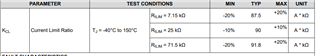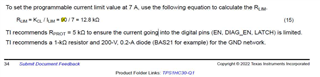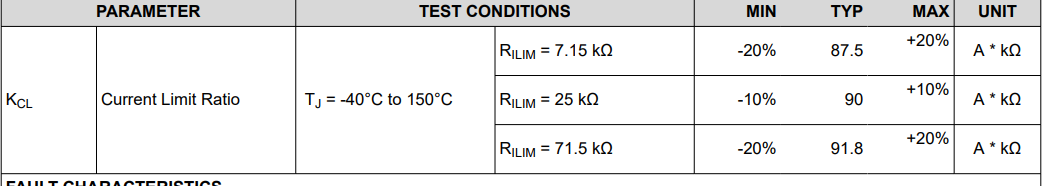If you have a related question, please click the "Ask a related question" button in the top right corner. The newly created question will be automatically linked to this question.

# TPS1HC30-Q1: How to Determine RILIMT for Current Limit

Part Number: TPS1HC30-Q1

Hi,

Question 1
ICL is described in 6.5 Electrical Characteristics of the datasheet. The ICL test conditions are divided into:
・RILIM = GND
・RILIM = open, or out of range
Also, the maximum and minimum values for ICL_LINPK and ICL_ENPS are specified by ICL. The ICL_ENPS test condition says RILIM=7.15KΩ to 71.5KΩ, but isn't the ICL condition RILIMT=GND or Open?

Question 2
There is a description of KCL in 6.5 Electrical Characteristics of the datasheet.
RILIM= KCL/ILIM, but what value should I use for KCL?
What is the reason for KCL=90 in the Typical Application in the datasheet?

Thanks,
Astro

• Hi Astro,

For question 1:

There are several configurations for current limit pin:

1) if connected with a resistor, the resistance needs to be between 7.15kohm to 71.5kohm, and the current limit is KCL/RLIM. KCL is determined in the following table. For any values in between, you can use linear interpolation.2) GND the ILIM pin will give you typical of 13A of current limt.

3) If the pin is open, or the resistance is not between 7.15k and 71.5k, then the typical will be 5.5A.

For question 2:

It should be an estimate of when Rlim is 25kohm, the KCL is 90 A*kohm. There will be variation if the resistance is different according to the table above.

Please let me know if this is clear.

Regards,

Yichi

• Hi, Yichi

It should be an estimate of when Rlim is 25kohm, the KCL is 90 A*kohm. There will be variation if the resistance is different according to the table above.

In the datasheet, we use KCL=90(A * kΩ) to determine RLIM. Any reason why?
I'm wondering if I can always assume KCL=90 for RLIN.
Also, you say that KCL can use linear interpolation, what do you mean?Thanks,

Astro

• Hi Astro,

The KCL is not a set number - it's Rlim dependent. As the range is close to 90, let's start with Kcl of 90. Say you want a current limit of 4A, 90/4 = 22.5kohm is roughly correct. Now, you can assume a linear function between 7.17kohm and 25kohm to have 87.5 to 90 Kcl, for 22.5kohm, Kcl is 89.6. Then the actual current limit is 89.6/22.5=3.98A. The example used 90 just as an approximation. Please let me know if you have further questions.Regards,

Yichi